Function Repository Resource:

# DeleteFrom

Delete an element from the value of a symbol and reset the symbol to the result

Contributed by: Ian Ford
 ResourceFunction["DeleteFrom"][x,n] deletes the element at position n in the value of x, and resets x to the result. If n is negative, the position is counted from the end. ResourceFunction["DeleteFrom"][x,{i,j,…}] deletes the part at position {i,j,…} from x. ResourceFunction["DeleteFrom"][x,{{i1,j1,…},{i2,j2,…}}] deletes parts at several positions.

## Details and Options

ResourceFunction["DeleteFrom"][x,pos] is equivalent to x=Delete[x,pos].
ResourceFunction["DeleteFrom"] has attribute HoldFirst.
Deleting the head of a particular element from x sets it equal to the result of applying FlattenAt to the value of x at that point.
Deleting position {} from x sets the value to Sequence[].
ResourceFunction["DeleteFrom"] works on SparseArray objects.
ResourceFunction["DeleteFrom"] works on associations, removing elements with the specified keys and returning them.

## Examples

### Basic Examples (3)

Assign x to a list:

 In:=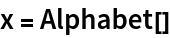Out=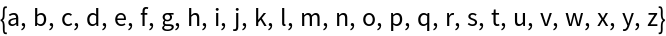Delete the element at position 3 from the list:

 In:=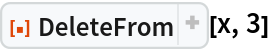Out=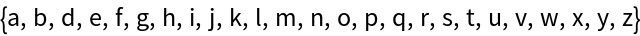The value assigned to x has changed:

 In:=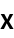Out=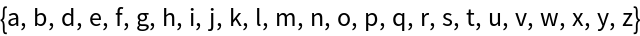Delete at position 2 from the end:

 In:=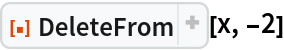Out=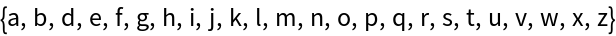Delete at positions 1 and 3:

 In:=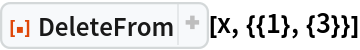Out=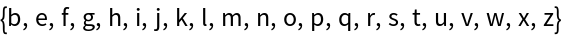Delete from a 2D array:

 In:=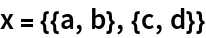Out=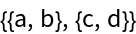In:=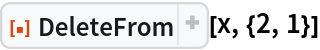Out=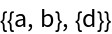Delete from an Association:

 In:=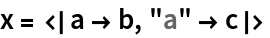Out=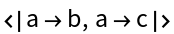In:=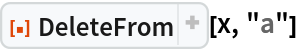Out=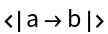In:=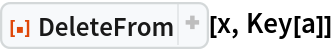Out=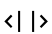### Scope (1)

DeleteFrom works on sparse arrays:

 In:=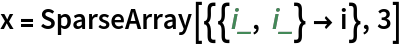Out=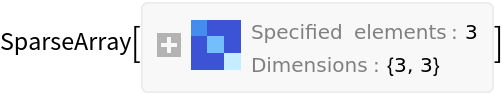In:=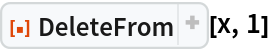Out=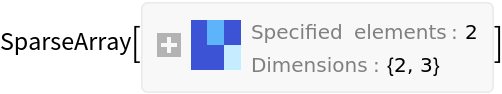### Properties and Relations (3)

 In:=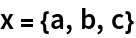Out=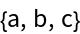In:=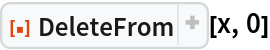Out=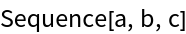Deleting at an empty list of positions does not change the value:

 In:=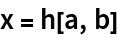Out=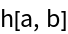In:=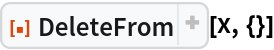Out=Deleting at position {} deletes the whole expression:

 In:=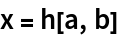Out=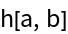In:=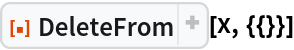Out=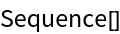## Requirements

Wolfram Language 11.3 (March 2018) or above

## Version History

• 1.0.0 – 17 December 2018